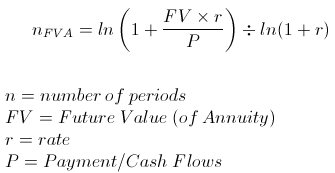# ln (Natural Log) in Python

## Question:

In this assignment I have completed all the problems except this one. I have to create a python script to solve an equation (screenshot).Unfortunately, in my research all over the internet I cannot figure out how in the world to either convert ln to log or anything usable, or anything. The code I have written so far is below. I will also post the answer that our teacher says we should get.

``````import math
p = 100
r = 0.06 / 12
FV = 4000

n = str(ln * ((1 + (FV * r) / p) / (ln * (1 + r))))

print ("Number of periods = " + str(n))
``````

The answer I should get is 36.55539635919235

Also, we are not using numpy. I already attempted that one.

Thanks!

`math.log` is the natural logarithm:

From the documentation:

math.log(x[, base]) With one argument, return the natural logarithm of
x (to base e).

``````n = math.log((1 + (FV * r) / p) / math.log(1 + r)))
``````

Note that in your code you convert n to a `str` twice which is unnecessary

Here is the correct implementation using numpy (`np.log()` is the natural logarithm)

``````import numpy as np
p = 100
r = 0.06 / 12
FV = 4000

n = np.log(1 + FV * r/ p) / np.log(1 + r)

print ("Number of periods = " + str(n))
``````

Output:

``````Number of periods = 36.55539635919235
``````
Categories: questions
Answers are sorted by their score. The answer accepted by the question owner as the best is marked with
at the top-right corner.